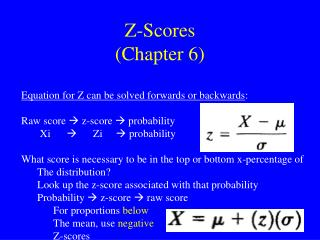DownloadDownload PresentationZ-Scores (Chapter 6)

# Z-Scores (Chapter 6)

Télécharger la présentation## Z-Scores (Chapter 6)

- - - - - - - - - - - - - - - - - - - - - - - - - - - E N D - - - - - - - - - - - - - - - - - - - - - - - - - - -
##### Presentation Transcript

1. Z-Scores(Chapter 6) • Equation for Z can be solved forwards or backwards: • Raw score  z-score  probability • Xi  Zi  probability • What score is necessary to be in the top or bottom x-percentage of • The distribution? • Look up the z-score associated with that probability • Probability  z-score  raw score • For proportions below • The mean, use negative • Z-scores

2. Finding The Proportion Between A Range Of Scores • Translate the raw scores to z-scores: • If the range spans the mean, add the areas in Column B • If the range is on one side of the mean, subtract the smaller area • From the larger area (using Column C) • Use Z-scores to find either the Proportion or the Probability

3. What Proportion OF The Population Has An IQ 90-110? μ = 100; σ = 15 • Area between mean (X=100, Z=0) and X=110 (Z=0.67) • Column B • Z=0.67 --> .2486 (Area =Probability) • Area between mean (X=100, Z=0) and X=90 (Z=-0.67) • Column B • Z=-0.67 --> .2486 (Area =Probability) • 0.2486 + 0.2486 = .4972 --> 49.72% (~50%) of Population

4. What Proportion OF The Population Has An IQ 70-90? μ = 100; σ = 15 • Area between mean (X=100, Z=0) and X=70 (Z=-2.00) • Column C • Z=-2.00 --> 0.0228 (Area =Probability/Proportion; 2.28%) • Area between mean (X=100, Z=0) and X=90 (Z=-0.67) • Column C • Z=-0.67 --> .2486 (Area =Probability/Proportion; 24.86%) • 0.2486 - 0.0228 = .2286 --> 22.86% of Population

5. Finding The Scores Which Define An Extreme (2-Tail) Group • What is the range of heart rates for 95% of the population? • μ = 71; σ = 9 • Upper ScoreLower Score • X = μ + Z * σ X = μ - Z * σ • = 71 + (1.96)*9 = 71 - (1.96)*9 • = 88.6 = 53.4